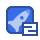# 达内 coreJava 习题答案

4星（超过85%的资源）24收藏

1，编写程序，判断给定的某个年份是否是闰年。 闰年的判断规则如下： （1）若某个年份能被4整除但不能被100整除，则是闰年。 （2）若某个年份能被400整除，则也是闰年。 import java.util.Scanner; class Bissextile{ public static void main(String[] arge){ System.out.print("请输入年份"); int year; //定义输入的年份名字为“year” Scanner scanner = new Scanner(System.in); year = scanner.nextInt(); if (year3000){ System.out.println("年份有误，程序退出！"); System.exit(0); } if ((year%4==0)&&(year%100!=0)||(year%400==0)) System.out.println(year+" is bissextile"); else System.out.println(year+" is not bissextile "); } } 2，给定一个百分制的分数，输出相应的等级。 90分以上 A级 80~89 B级 70~79 C级 60~69 D级 60分以下 E级 import java.util.Scanner; class Mark{ public static void main(String[] args){ System.out.println("请输入一个分数"); //定义输入的分数为“mark”，且分数会有小数 double mark; Scanner scanner = new Scanner(System.in); mark = scanner.nextDouble(); //判断是否有输入错误。 if(mark100){ System.out.println("输入有误！ "); System.exit(0); } /*判断分数的等级 90分以上者A级， 80~89分者 B级，70~79分者 C级， 60~69者 D级，60分以下 E级 */ if (mark>=90) System.out.println("this mark is grade \'A\' "); else if (mark>=80) System.out.println("this mark is grade \'B\' "); else if (mark>=70) System.out.println("this mark is grade \'C\' "); else if (mark>=60) System.out.println("this mark is grade \'D\' "); else System.out.println("this mark is grade \'E\' "); } } 3，编写程序求 1+3+5+7+……+99 的和值。 class he{ public static void main(String[] args){ int number = 1; //初始值1，以后再+2递增上去 int sum = 0; for ( ; number <100; number+=2 ){ sum += number; } System.out.println("1+3+5+7+……+99= " +sum); } } 4、利用for循环打印 9*9 表? 1*1=1 1*2=2 2*2=4 1*3=3 2*3=6 3*3=9 1*4=4 2*4=8 3*4=12 4*4=16 1*5=5 2*5=10 3*5=15 4*5=20

...展开详情

2014-08-08

2013-08-27

2013-02-21

• 8
资源
• 0
粉丝
•等级达内 coreJava 习题答案 22积分/C币 立即下载
1/0